# Applied Statistics 101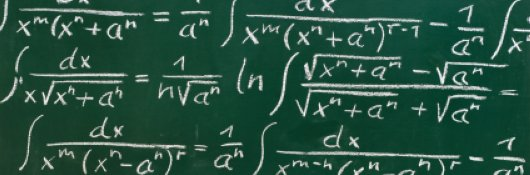\$70.00
no certificate
- OR -
\$95.00
with CEU Certificate*
Start Right Now!
Taking multiple courses? Save with our platinum program.

• 14
Lessons
• 17
Exams &
Assignments
• 1,323
Students
have taken this course
• 21
Hours
average time
• 2.1
CEUs

## Course Description

Large sets of numbers can be daunting, and characterizing them in a few words or numbers can be even more daunting. This course considers how to take data sets--whether large or small--and describe them using a few numbers (descriptive statistics). This, however, is only a small portion of the course.

The majority of the course is dedicated to reaching statistically justified conclusions on the basis of these descriptive statistics. For instance, does the average value of one data set deviate from another in what might be called a "statistically significant" manner? To this end, the course covers cross tabulation of data (including the chi-square test), correlation, linear regression, Student's t-tests, analysis of variance (ANOVA), repeated measures analysis, and factor analysis.

Although the course does not always provide rigorous mathematical justifications for every aspect of the statistical tools and theory discussed therein, it does provide common-sense explanations of many of these aspects, and it includes numerous real-world examples to illustrate the use of these tools.

Thus, this course teaches students to take sets of data, describe them using a few numbers (including the mean, variance, and skewness), and then reach statistically justifiable conclusions about those data sets. Students should come away from the course with confidence in their ability to tackle basic applied statistics problems and with the fundamental knowledge needed to learn more in-depth statistical theory.

• Completely Online
• Self-Paced
• Printable Lessons
• Full HD Video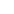• 6 Months to Complete
• Start Anytime
• PC & Mac Compatible
• Android & iOS Friendly
• Accredited CEUs
•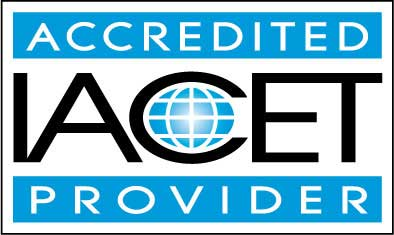## Course Lessons

Average Lesson Rating: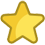#### Lesson 1 - Descriptive Statistics I

o Review arithmetic means and some associated concepts in descriptive statistics
o Consider other types of means, including geometric and power means
11 Total Points
•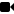Lesson 1 Video A
•Lesson 1 Video B : How to Take an Exam
•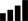Take Survey: Reasons for Taking this Course
•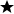Complete Assignment: Introduction
•Complete Exam: Lesson 1 Descriptive Statistics I
•Complete: Feedback about the exam.

#### Lesson 2 - Descriptive Statistics II

o Use moments about the mean to represent measures of dispersion and asymmetry
o Calculate the variance, standard deviation, and skewness of data sets
10 Total Points
•Lesson 2 Video
•Complete Exam: Lesson 2 Descriptive Statistics II

#### Lesson 3 - Frequencies

o Create frequency tables to represent data sets
o Calculate relative, cumulative, and cumulative relative frequencies
o Create and interpret bar graphs and histograms
9 Total Points
•Lesson 3 Video
•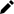Review Practice Worksheet: Example2Explainedfurther.docx
•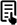Review Article: Variance Formulas in Excel
•Complete Exam: Lesson 3 Frequencies

#### Lesson 4 - Multivariate Data

o Recognize multivariate data versus univariate data
o Represent multivariate data using tables and scatterplots
o Calculate descriptive statistics for multivariate data, including covariances
10 Total Points
•Lesson 4 Video
•Complete Exam: Lesson 4 Multivariate Data

#### Lesson 5 - Cross Tabulation I

o Create cross tabulations for bivariate data sets
o Review the basic terminology and procedure associated with statistical hypothesis testing
10 Total Points
•Lesson 5 Video
•Review Article: Contingency table
•Complete Exam: Lesson 5 Cross Tabulation I

#### Lesson 6 - Cross Tabulation II

o Understand how to calculate the chi-square statistic for a cross tabulation
o Use the chi-square statistic to test hypotheses regarding cross tabulations
10 Total Points
•Lesson 6 Video
•Complete Exam: Lesson 6 Cross Tabulation II

#### Lesson 7 - Correlation

o Understand how variance can be used to define a statistic that measures the linear relationship between variables
o Use the correlation coefficient to quantify the correlation between two variables
10 Total Points
•Lesson 7 Video
•Review Article: Correlation
•Complete Exam: Lesson 7 Correlation

#### Lesson 8 - Student's t-Tests I

o Learn and apply hypothesis testing procedure to the one-sample Student's t-test 10 Total Points
•Lesson 8 Video
•Complete Exam: Lesson 8 Students t-Tests I

#### Lesson 9 - Student's t-Tests II

o Apply the paired two-sample Student's t-test to determine if two samples have statistically different means 10 Total Points
•Lesson 9 Video
•Review Article: Making Sense of the Two-Sample T-Test
•Complete Exam: Lesson 9 Students t-Tests II

#### Lesson 10 - One-Way ANOVA

o Identify the test statistic for one-way ANOVA
o Use one-way ANOVA to compare the means of multiple sample groups
10 Total Points
•Lesson 10 Video
•Review Article: A table of values for the F distribution
•Complete Exam: Lesson 10 One-Way ANOVA

#### Lesson 11 - Repeated Measures

Recognize repeated measures designs
Determine an alternate method of evaluating means for repeated measures designs (repeated measures ANOVA)
10 Total Points
•Lesson 11 Video
•Review 2 Articles: Repeated Measures ANOVA; Total Sum of squares
•Complete Exam: Lesson 11 Repeated Measures

#### Lesson 12 - Factor Analysis

o Recognize some of the key terms associated with factor analysis
o Understand the overall purpose and procedures of exploratory and confirmatory factor analysis
10 Total Points
•Lesson 12 Video
•Complete Exam: Lesson 12 Factor Analysis

#### Lesson 13 - Linear Regression I

Previously, we considered scatterplots as a way to display bivariate data sets. Scatterplots lead us to ask whether we can statistically construct a function that approximates the data. 10 Total Points
•Lesson 13 Video
•Complete Exam: Lesson 13 Linear Regression I

#### Lesson 14 - Linear Regression II

We now extend our look at linear regression by considering path diagrams, which can be a useful tool for visually representing the relationships among variables in a data set. 96 Total Points
•Lesson 14 Video
•Take Survey: Program Evaluation Follow-up Survey (End of Course)
•Complete Exam: Lesson 14 Linear Regression II
•Complete: The Final Exam
226
Total Course Points

## Learning Outcomes

By successfully completing this course, students will be able to:
• Define descriptive statistics.
• Demonstrate statistics and the use frequencies and how to solve these problems.
• Identify multivariate data.
• Demonstration Cross Tabulation problems and solutions
• Demonstrate understanding of correlation in statistics.
• Demonstrate problem and solution use of t-Tests.
• Demonstrate One-Way ANOVA statistical problems and solutions
• Demonstrate Repeated Measures.
• Demonstrate Factor Analysis.
• Describe basic usage of SPSS for graphing and solving applied statistics problems, and
• Demonstrate mastery of lesson content at levels of 70% or higher.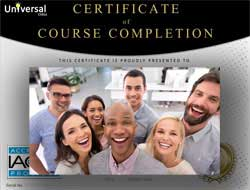• Document Your Lifelong Learning Achievements
• Earn an Official Certificate Documenting Course Hours and CEUs
• Verify Your Certificate with a Unique Serial Number Online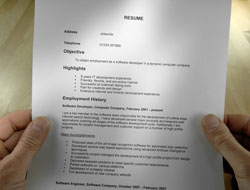Course Title: Applied Statistics 101
Course Number: 9770454
Course Requirements: View Course Requirements
Lessons Rating: 4.8 / 5 Stars (421 votes)
Languages: English - United States, Canada and other English speaking countries
Category:
Course Type: General Education (Self-Paced, Online Class)
CEU Value: 2.1 IACET CEUs (Continuing Education Units)
CE Accreditation: Universal Class, Inc. has been accredited as an Authorized Provider by the International Association for Continuing Education and Training (IACET).
Assessment Method: Lesson assignments and review exams
Instructor: April Cordry-Moore
Syllabus: View Syllabus
Duration: Continuous: Enroll anytime!
Course Fee: \$70.00 (no CEU Certification) || with Online CEU Certification: \$95.00

Course Only
One Course
No Certificate / No CEUs
\$70
for 6 months

Billed once
This course only
 Includes certificate X Includes CEUs X Self-paced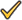Instructor supportTime to complete 6 months No. of courses 1 course
Certificate Course
One Course
Certificate & CEUs
\$95
for 6 months

Billed once
This course only
 Includes certificateIncludes CEUsSelf-pacedInstructor supportTime to complete 6 months No. of courses 1 course
Platinum Yearly
ALL COURSES
Certificates & CEUs
\$189
per year

Billed once
Includes all 500+ courses
 Includes certificateIncludes CEUsSelf-pacedInstructor supportTime to complete 12 Months No. of courses 500+
Platinum 2 Years
ALL COURSES
Certificates & CEUs
\$299
for 2 years
You save \$79.00!
Billed once
Includes all 500+ courses
 Includes certificateIncludes CEUsSelf-pacedInstructor supportTime to complete 24 Months No. of courses 500+

## Student Testimonials

• "The instructor always got back to me quickly when I had a question or comment. He also graded the assignments and exams in a very timely manner." -- Cindy C.